# Real Estate Finance Study Set 1

## Quiz 4 : Fixed Interest Rate Mortgage LoansStudy FlashcardsLooking for Finance Homework Help?

## Quiz 4 :Fixed Interest Rate Mortgage Loans

Question TypeWhat is the connection between the Truth-in-Lending Act and the annual percentage rate (APR)
Free
Essay

The Federal Truth-in-Lending Act (FTL) is a legislation which makes it mandatory for lenders to make uniform disclosure of financial information contained in loan agreements. This disclosure will help borrowers to make comparison of cost of different loan agreements. The FTL legislation requires that three days after a mortgage application is made by the borrower, the lender must make the disclosures.
A very important disclosure is about the Annual Percentage Rate (APR) being charged on the loan. The FTL guidelines require accurate disclosure of the APR. Rounding of the APR to the nearest one-quarter of a percent is allowed as long as it falls within the nearest one-eighth of a percent of the APR computed as per the method prescribed in the FTL.
The legislation also requires disclosure of change in market interest rates and its effect on APR from the time of application until making of the loan. The lender must make prior additional disclosures to the borrower before making such loan. Thus, borrower will get to know the actual interest to be charged on loan before taking the loan.

Tags
Choose question tagQualify is applying for a $100,000 GPM loan for 25 years at an interest rate of 9 percent. Payments would be designed so as to graduate at the rate of 7.5 percent for three years beginning with payments in the second year. a. What would monthly payments be for Mr. Qualify in each of the first five years of the loan b. What would the loan balance be on the GPM at the end of year 3 c. If the lender charged 4 points at origination, what would be the effective interest rate on this loan after five years Free Essay Answer: Answer: Introduction: Constant payment mortgage loan (CPM) is the payment pattern used in the real estate for financing residential properties. Constant payment mortgage is used primarily for financing apartment, complexes, and shopping centres. a. Computation of payments at the beginning of year: The initial monthly payment will be kept at$498.57. The payments at the beginning of year 2, 3, 4, and 5 will be computed with the help of excel.
Following are the formulas entered in excel to compute the payments at the beginning of the period.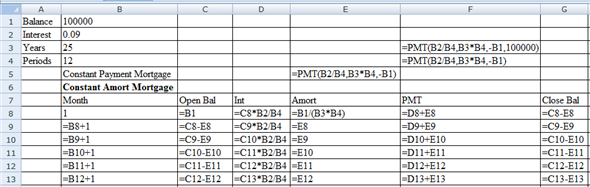Following is the results of the above entered formulas in excel: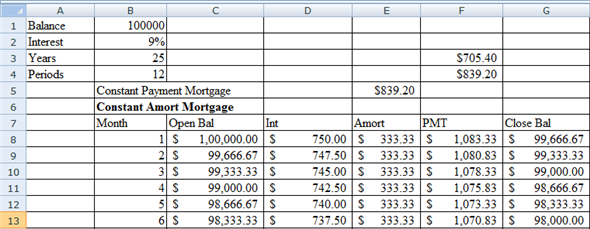The monthly payments for year 1, 2, 3, 4, and 5 will be $1,083.33,$1,080.83, $1,078.33,$1,075.83 and $1,073.33 respectively. b. Computation of payment under GPM (graduated payment mortgage): The payments for the different years under GPM will be computed with the help of excel. Following are the formulas entered in excel to compute the loan balances: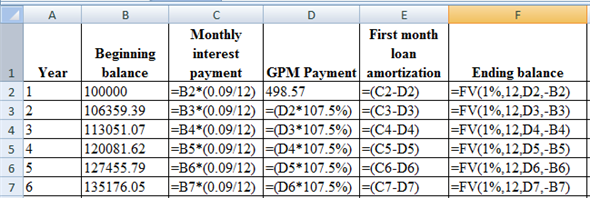Following is the results of the above entered formulas in excel: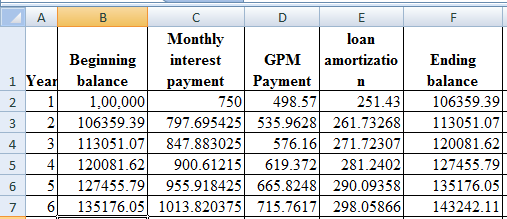Therefore the balance at the end of year three will be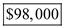c. The interest rate on the loan is 9 percent. The loan originated with four discount points.Hence, the effective yield will be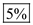Tags Choose question tagA fully amortizing mortgage loan is made for$100,000 at 6 percent interest for 20 years. a. Calculate the monthly payment for a CPM loan. b. What will the total of payments be for the entire 20-year period Of this total, how much will be interest c. Assume the loan is repaid at the end of 8 years. What will be the outstanding balance How much total interest will have been collected by then d. The borrower now chooses to reduce the loan balance by $5,000 at the end of year 8. (1) What will be the new loan maturity assuming that loan payments are not reduced (2) Assume the loan maturity will not be reduced. What will the new payments be Free Essay Answer: Answer: (a) Determine the monthly payment: Monthly payment is the amount to be paid for the loan repayment. As a text book notation, financial calculator should be used to calculate the monthly payment (PMT). It is given that the borrower obtains a loan of$100,000 at 6% interest for 20 years. These annual details should be converted into monthly details by dividing the interest with 12 and the number of periods will be 240 (20 years × 12).
The following inputs should be given to the financial calculator to derive the monthly payment:With the help of the financial calculator or spreadsheet, the monthly payment (PMT) can be determined as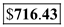.
(b) Determine the total of payments for the entire 20-year period and the interest portion:
It is calculated that the monthly payment will be $716.43. Number of periods is 240. Calculate the total of payments as follows:Hence, the total amount payable for 240 periods will be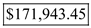. Among this total payment,$100,000 will be the principal portion and the balance of $171,943.45 ($271,943.35 - $100,000) would be the interest payment. (c) Determine the amount of outstanding loan if the loan is repaid at the end of 8 years: It is given that the borrower obtains a loan of$100,000 at 6% interest. If the loan is repaid at the end of 8 years, calculate the present value of future payments for the remaining 12 years of out 20 years' time period of the loan.
Total number of periods will be 144 (12 years × 12). The monthly interest rate will remain at 0.50%.
The following inputs should be given to the financial calculator to derive the monthly payment: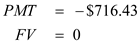Solve for amount:
With the help of the financial calculator, the present value can be determined as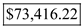The amount of interest paid is the difference between the principal portion and the sum of total payments made and the mortgage balance at the end of 8 years calculated as below:Hence, the total interest collected will be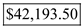.
Calculate the principal paid as follows:Therefore, the principal payment is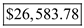(d) (1) Determine the new loan maturity balance assuming that loan payments are not made:
First determine the balance of loan after deducting $5,000 payment. In this regard, determine the present value of loan at the end of 8 th year (remaining years 12 years). The rate of interest per month is 0.5% and the number of period will be 144 (12 × 12 years). Provide the following inputs to determine the present value of the remaining loan at the end of year 8:Solve for payment: With the help of the financial calculator, the present value can be determined asNow determine the outstanding balance after the payment of$5,000 as below: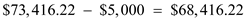Now, determine the number of periods for the new loan maturity with the interest rate of 0.5%, monthly payment as $716.43 and the present value of loan as$68,416.22. Input these details in the financial calculator to determine the number of periods as below:Solve for maturity:
Using the financial calculator, the number of periods can be determined as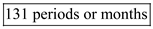.
(2) Determine the new monthly payment assuming loan maturity will not be reduced:
If the loan maturity will not be reduced, the number of periods will remain at 144 periods (12 × 12 years), rate of interest per month is 0.5%, and the present value of loan is $68,416.22. Input these details in the financial calculator to determine the monthly payment as below:Solve for payment: Using the financial calculator, the monthly payment can be determined as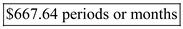. Note: Rounding off used therefore, answers calculate by others might be different in fractions. Tags Choose question tagAs inflation increases, the impact of the tilt effect is said to become even more burdensome on borrowers. Why is this so Essay Answer: Tags Choose question tagWhy do lenders charge origination fees, especially loan discount fees Essay Answer: Tags Choose question tagA 30-year fully amortizing mortgage loan was made 10 years ago for$75,000 at 6 percent interest. The borrower would like to prepay the mortgage balance by $10,000. a. Assuming he can reduce his monthly mortgage payments, what is the new mortgage payment b. Assuming the loan maturity is shortened and using the original monthly payments, what is the new loan maturity Essay Answer: Tags Choose question tagIn the absence of loan fees , does repaying a loan early ever affect the actual or true interest cost to the borrower Essay Answer: Tags Choose question tagWhat are loan closing costs How can they be categorized Which of the categories influence borrowing costs and why Essay Answer: Tags Choose question tagA fully amortizing mortgage loan is made for$80,000 at 6 percent interest for 25 years. Payments are to be made monthly. Calculate: a. Monthly payments. b. Interest and principal payments during month 1. c. Total principal and total interest paid over 25 years. d. The outstanding loan balance if the loan is repaid at the end of year 10. e. Total monthly interest and principal payments through year 10. f. What would the breakdown of interest and principal be during month 50
Essay
Tags
Choose question tagWhy do the monthly payments in the beginning months of a CPM loan contain a higher proportion of interest than principal repayment
Essay
Tags
Choose question tagDefine amortization.List the five types discussed in this chapter.
Essay
Tags
Choose question tagWhy do level or constant monthly mortgage payments increase so sharply during periods of inflation What does the tilt effect have to do with this
Essay
Tags
Choose question tagExcel. Refer to the "Ch4 GPM" tab in the Excel Workbook provided on the Web site. How would the initial payment change if the payments increase by 5 percent each year instead of 7.5 percent
Essay
Tags
Choose question tagA fully amortizing mortgage loan is made for $100,000 at 6 percent interest for 30 years. Determine payments for each of the periods a - d below if interest is accured: a. Monthly. b. Quarterly. c. Annually. d. Weekly. Essay Answer: Tags Choose question tagA property is purchased for$70,000. The purchase is financed with a GPM carrying a 12 percent interest rate. A 7.5 percent rate of graduation will be applied to monthly payments beginning each year after the loan is originated for a period of five years. The initial loan amount is $63,000 for a term of 30 years. The homeowner expects to sell the property after seven years. a. If the initial monthly payment is$498.57, what will payments be at the beginning of years 2, 3, 4, and 5 b. What would the payment be if a CPM loan was available c. Assume the loan is originated with two discount points. What is the effective yield on the GPM
Essay
Tags
Choose question tagA borrower obtains a fully amortizing CPM loan for $125,000 at 11 percent interest for 10 years. What will be the monthly payment on the loan If this loan had a maturity of 30 years, what would be the monthly payment Essay Answer: Tags Choose question tagA fully amortizing mortgage is made for$80,000 for 25 years. Total monthly payments will be $900 per month. What is the interest rate on the loan Essay Answer: Tags Choose question tagWhat are the major differences between the CAM, and CPM loans What are the advantages to borrowers and risks to lenders for each What elements do each of the loans have in common Essay Answer: Tags Choose question tagRegarding Problem 3, how much total interest and principal would be paid over the entire 30-year life of the mortgage in each case Which payment pattern would have the greatest total amount of interest over the 30-year term of the loan Why (Reference Problem 3) A fully amortizing mortgage loan is made for$100,000 at 6 percent interest for 30 years. Determine payments for each of the periods a - d below if interest is accured: a. Monthly. b. Quarterly. c. Annually. d. Weekly.
EssayA fully amortizing mortgage is made for $100,000 at 6.5 percent interest. If the monthly payments are$1,000 per month, when will the loan be repaid